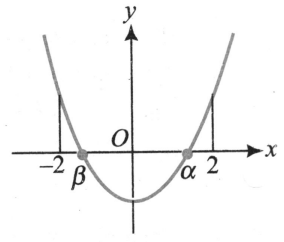Theory of expressions
Question

# If roots of ,and 4a + 2b + c > 0, 4a - 2b + c > 0, and c < 0, then possible value/values of $\left[\mathrm{\alpha }\right]+\left[\mathrm{\beta }\right]$ is/are (where [.] represents greatest integer function)

Moderate
Solution

## From the given conditions we have following possible graph of y = f(x)From the graph,

Get Instant Solutions